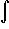#Interactive Real Analysis

Next | Previous | Glossary | Map

## 7.4. Lebesgue Integral

### Example 7.4.9(a): Riemann implies Lebesgue Integrable

Find the Lebesgue integral of x cos(x) over the interval [-1, 1].
The function x cos(x) is continuous over the interval [-1, 1]. Therefore it is Riemann integrable. By the previous theorem it must then be Lebesgue integrable and the two integrals agree. Therefore we can compute the Riemann integral, for which we have techniques such as integration by parts:

Let g'(x) = cos(x), f(x) = x and G(x) = x sin(x). Using integration by parts with a = -1 and b = 1 we have:x cos(x) = G(b) - G(a) -sin(x) =
= b sin(b) - a sin(a) + (cos(b) - cos(a)) =
= sin(1) + sin(-1) + (cos(1) - cos(-1)) = 0
Therefore the Lebesgue integral[-1, 1] x cos(x) = 0
as well.

Note that we have shown in a prior example that the (Riemann) integral of an odd function over an interval [-a, a] is always zero. Since x cos(x) is odd, the integral must therefore be zero by that example.

Next | Previous | Glossary | Map